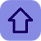# What Is Object Oriented Programming Lanugages

Posted by Tushar Bedekar
There are basically two eras of the programming languages to which we have seen which are as follow:-

• Structured (procedure) oriented programming language
• Object oriented programming language
There are many structure oriented programming languages such as C, Cobol, Pascal, Assembly languages etc. where we make use of functions (procedures) and supposed to write the programme sequentially with the data and functions mashed up in the form of the sequential statements.
Here in the procedures oriented language the data is allowed to flow freely in the programme.A confusing statement. To understand it let's take an example we have a programme which consist of global variable declaration and being assessed by the two or more number of functions as given bellow

#include<stdio.h>
int a=10;

{
int b=5;
a=a+b;
}

int sum()
{
int c=7;
a=a+b;
printf("%d",a);
}

int main()
{
sum();
return 0;
}

as here we are seeing that the different functions sum() and add() are modifying the value of the (global variable a).Also as the function sum() is being executed after add().So due to which the value of variable a gets changed before the execution of sum(). This actually the free flow of the data within the different functions.

This drawback is being removed by the object-oriented programming language which binds the data and functions together. This data and function bounded together was collectively known as object.